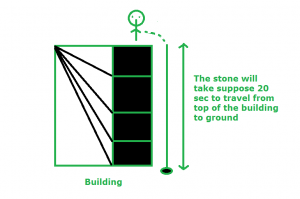# Puzzle | Height of Building

• Difficulty Level : Basic
• Last Updated : 30 Dec, 2017

There is man on the terrace of a building and he wants to find the height of the building. Man wears a watch only and does not have any instruments to measure anything. There are also some stones on the terrace.
How can he find the height of the building from terrace ?

Solution: The man can find height of building by using stone and watch. First, he will throw stone from terrace and note the time, the stone is taking to reach the ground.Since, we have the time taken by stone to reach the ground, we can find the height of the building by the formula:
h = ut + 1/2gt^2

where,
h = height
t = time (we calculated it already)
u = initial velocity(Initial velocity will be 0)
g = gravitational acceleration i.e. 9.8 m/s2

Assume the time taken by stone to reach ground is 20 sec. Man can find the height of the building by putting it in the formula:

h = u * t + 1/2 * g * t^2
= 0 * 20 + 1/2 * 9.8 * (20)^2
= 1960 meter

My Personal Notes arrow_drop_up